Next: RCL Circuit Up: Circuit Equations Previous: RL Circuit

## LC Circuit

Lets now consider the LC circuit in figure 2.3 which has no resistive element.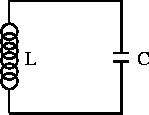Figure 2.3:  LC circuit.

Kirchoff's voltage law applied to the loop is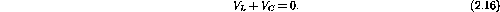Substituting our previous expressions for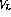and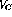givesUsing I = dQ/dt gives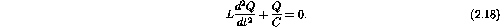The circuit equation is second-order in Q and one possible solution is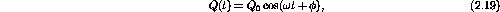where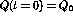is the initial charge on the capacitor andis an arbitrary phase constant. Considering the cases of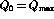, gives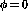. The angular frequencyis totally determined by the other parameters of the circuit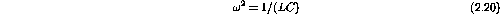and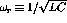is the natural or resonance frequency of the circuit.

We can also solve for the current and voltage across the capacitor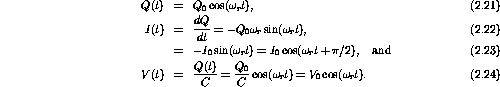Notice that unlike the transient current and voltage responses of the RC and RL circuits, the LC circuit oscillates. The energy in the circuit is shared back and forth between the inductor and capacitor.

Doug Gingrich
Tue Jul 13 16:55:15 EDT 1999